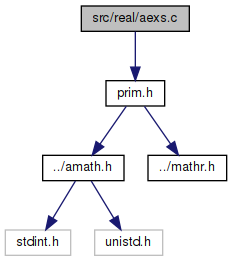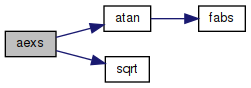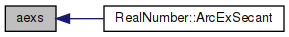amath  1.8.5 Simple command line calculator
aexs.c File Reference

Inverse excosecant function. More...

`#include "prim.h"`
Include dependency graph for aexs.c:Go to the source code of this file.

## Functions

double aexs (double x)
Inverse exsecant function. More...

## Detailed Description

Inverse excosecant function.

Inverse exsecant function.

Definition in file aexs.c.

## ◆ aexs()

 double aexs ( double x )

Inverse exsecant function.

```Method
aexsec(x) = arcsec(x+1)
= arccos(1/(x+1))
= arctan(sqrt(x^2+2*X))
```

Definition at line 47 of file aexs.c.

References atan(), and sqrt().

Referenced by RealNumber::ArcExSecant().

48 {
49  double a, b, c;
50
51  if (x > -2.0 && x < 0.0)
52  return NAN;
53
54  a = x * x + 2 * x;
55  b = sqrt(a);
56  c = atan(b);
57  return c;
58 }
double atan(double x)
Inverse tangent function.
Definition: atan.c:103
#define NAN
Definition: mathr.h:53
double sqrt(double x)
Square root function.
Definition: sqrt.c:119
Here is the call graph for this function:Here is the caller graph for this function: